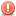##Elliptical 3D camera orbit- really need help with the math, please

Heres my issue- Id have a 3D camera rotating around its target. I want its rotation around the Y-axis (yaw) to be elliptical.Ive been advised by others to change The location of the camera over time with a varying distance instead of a static one. Im not really sure how to go about thisAny help would be a life-saver.

This is the code for the camera:

Actionscript Code:
`public override function orbit(pitch:Number, yaw:Number, useDegrees:Boolean=true, target:DisplayObject3D=null):void        {            target = target || _target;            target = target || DisplayObject3D.ZERO;            if(useDegrees)            {                pitch *= (Math.PI/180);                yaw *= (Math.PI/90);            }                       // Number3D.sub            var dx             :Number = target.world.n14 - this.x;            var dy             :Number = target.world.n24 - this.y;            var dz             :Number = target.world.n34 - this.z;                       // Number3D.modulo            var distance     :Number = Math.sqrt(dx*dx+dy*dy+dz*dz);            // Rotations            var rx :Number = Math.cos(yaw) * Math.sin(pitch);            var rz :Number = Math.sin(yaw) * Math.sin(pitch);            var ry :Number = Math.cos(pitch);                       // Move to specified location            this.x = target.world.n14 + (rx * distance);            this.y = target.world.n24 + (ry * distance);            this.z = target.world.n34 + (rz * distance);                       this.lookAt(target);        }`